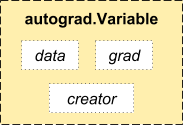Autograd is now a core torch package for automatic differentiation. It uses a tape based system for automatic differentiation.

In the forward phase, the autograd tape will remember all the operations it executed, and in the backward phase, it will replay the operations.

## Variable¶

In autograd, we introduce a Variable class, which is a very thin wrapper around a Tensor. You can access the raw tensor through the .data attribute, and after computing the backward pass, a gradient w.r.t. this variable is accumulated into .grad attribute.Variable

There’s one more class which is very important for autograd implementation - a Function. Variable and Function are interconnected and build up an acyclic graph, that encodes a complete history of computation. Each variable has a .grad_fn attribute that references a function that has created a function (except for Variables created by the user - these have None as .grad_fn).

If you want to compute the derivatives, you can call .backward() on a Variable. If Variable is a scalar (i.e. it holds a one element tensor), you don’t need to specify any arguments to backward(), however if it has more elements, you need to specify a grad_output argument that is a tensor of matching shape.

import torch
print(x)  # notice the "Variable containing" line

print(x.data)

print(x.grad)

print(x.grad_fn)  # we've created x ourselves


Do an operation of x:

y = x + 2
print(y)


y was created as a result of an operation, so it has a grad_fn

print(y.grad_fn)


More operations on y:

z = y * y * 3
out = z.mean()

print(z, out)


let’s backprop now and print gradients d(out)/dx

out.backward()


By default, gradient computation flushes all the internal buffers contained in the graph, so if you even want to do the backward on some part of the graph twice, you need to pass in retain_variables = True during the first pass.

x = Variable(torch.ones(2, 2), requires_grad=True)
y = x + 2
y.backward(torch.ones(2, 2), retain_graph=True)
# the retain_variables flag will prevent the internal buffers from being freed

z = y * y
print(z)


gradient = torch.randn(2, 2)